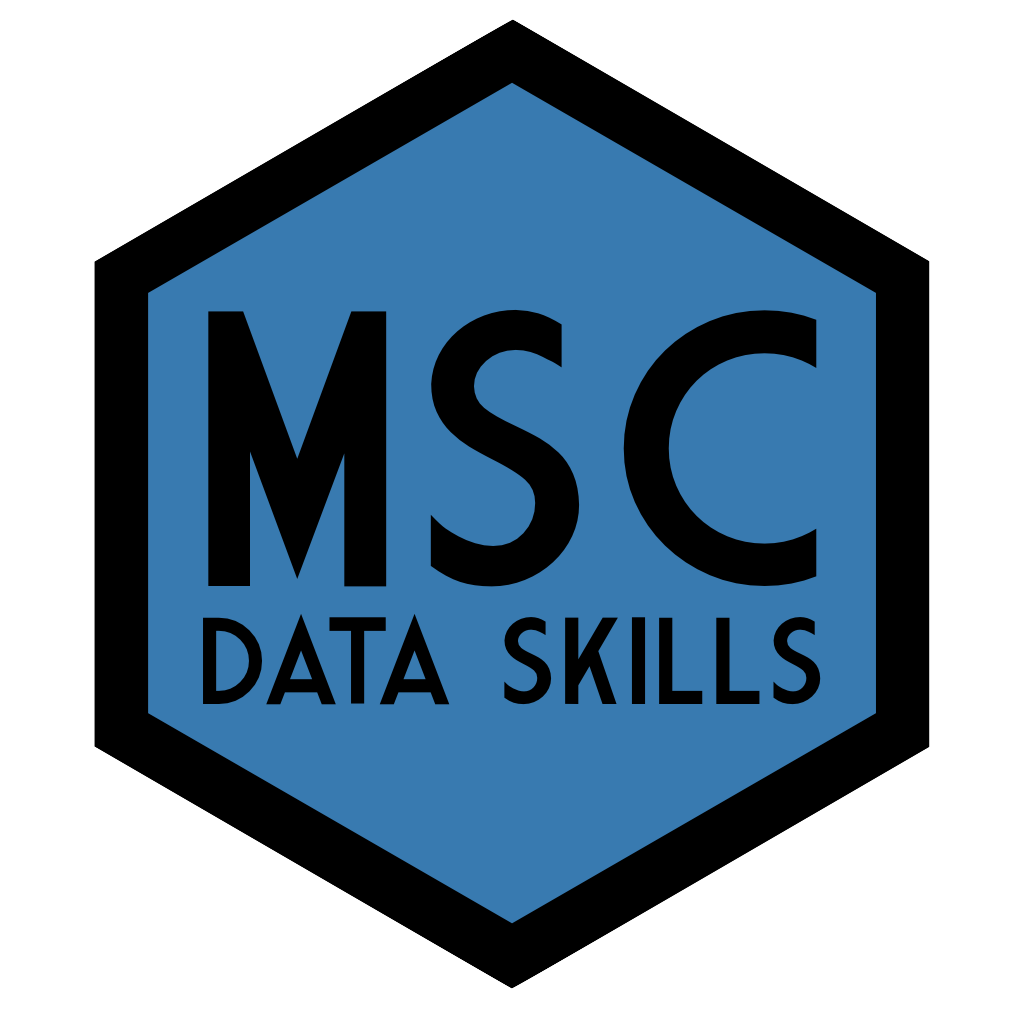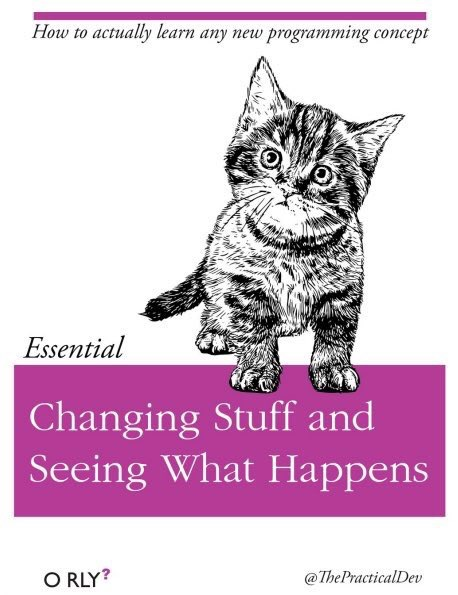# OverviewThis course provides an overview of skills needed for reproducible research and open science using the statistical programming language R. Students will learn about data visualisation, data tidying and wrangling, archiving, iteration and functions, probability and data simulations, general linear models, and reproducible workflows. Learning is reinforced through weekly assignments that involve working with different types of data.

## 0.1 Course Aims

This course aims to teach students the basic principles of reproducible research and to provide practical training in data processing and analysis in the statistical programming language R.

## 0.2 Intended Learning OutcomesBy the end of this course students will be able to:

• Write scripts in R to organise and transform data sets using best accepted practices
• Explain basics of probability and its role in statistical inference
• Critically analyse data and report descriptive and inferential statistics in a reproducible manner

## 0.3 Course Resources

• Data Skills Videos Each chapter has several short video lectures for the main learning outcomes at the playlist . The videos are captioned and watching with the captioning on is a useful way to learn the jargon of computational reproducibility. If you cannot access YouTube, the videos are available on the course Teams and Moodle sites or by request from the instructor.

• dataskills This is a custom R package for this course. You can install it with the code below. It will download all of the packages that are used in the book, along with an offline copy of this book, the shiny apps used in the book, and the exercises.

devtools::install_github("psyteachr/msc-data-skills")
• glossary Coding and statistics both have a lot of specialist terms. Throughout this book, jargon will be linked to the glossary.

## 0.4 Course Outline

The overview below lists the beginner learning outcomes only. Some lessons have additional learning outcomes for intermediate or advanced students.

1. Getting Started
1. Understand the components of the RStudio IDE
2. Type commands into the console
3. Understand function syntax
4. Install a package
5. Organise a project
6. Create and compile an Rmarkdown document
2. Working with Data
2. Import data from CSV and Excel files
3. Create a data table
4. Understand the use the basic data types
5. Understand and use the basic container types (list, vector)
6. Use vectorized operations
7. Be able to troubleshoot common data import problems
3. Data Visualisation
1. Understand what types of graphs are best for different types of data
2. Create common types of graphs with ggplot2
3. Set custom labels, colours, and themes
4. Combine plots on the same plot, as facets, or as a grid using cowplot
5. Save plots as an image file
4. Tidy Data
1. Understand the concept of tidy data
2. Be able to convert between long and wide formats using pivot functions
3. Be able to use the 4 basic tidyr verbs
4. Be able to chain functions using pipes
5. Data Wrangling
1. Be able to use the 6 main dplyr one-table verbs: select(), filter(), arrange(), mutate(), summarise(), group_by()
2. Be able to wrangle data by chaining tidyr and dplyr functions
3. Be able to use these additional one-table verbs: rename(), distinct(), count(), slice(), pull()
6. Data Relations
1. Be able to use the 4 mutating join verbs: left_join(), right_join(), inner_join(), full_join()
2. Be able to use the 2 filtering join verbs: semi_join(), anti_join()
3. Be able to use the 2 binding join verbs: bind_rows(), bind_cols()
4. Be able to use the 3 set operations: intersect(), union(), setdiff()
7. Iteration & Functions
1. Work with iteration functions: rep(), seq(), and replicate()
2. Use map() and apply() functions
3. Write your own custom functions with function()
4. Set default values for the arguments in your functions
8. Probability & Simulation
1. Generate and plot data randomly sampled from common distributions: uniform, binomial, normal, poisson
2. Generate related variables from a multivariate distribution
3. Define the following statistical terms: p-value, alpha, power, smallest effect size of interest (SESOI), false positive (type I error), false negative (type II error), confidence interval (CI)
4. Test sampled distributions against a null hypothesis using: exact binomial test, t-test (1-sample, independent samples, paired samples), correlation (pearson, kendall and spearman)
5. Calculate power using iteration and a sampling function
9. Introduction to GLM
1. Define the components of the GLM
2. Simulate data using GLM equations
3. Identify the model parameters that correspond to the data-generation parameters
4. Understand and plot residuals
5. Predict new values using the model
6. Explain the differences among coding schemes
10. Reproducible Workflows
1. Create a reproducible script in R Markdown
3. Include a table
4. Include a figure
5. Use source() to include code from an external file
6. Report the output of an analysis using inline R

## 0.5 Formative Exercises

Exercises are available at the end of each lesson’s webpage. These are not marked or mandatory, but if you can work through each of these (using web resources, of course), you will easily complete the marked assessments.